# Writing and Graphing Inequalities

##By Anywhere Math# Writing and Graphing Inequalities (6.EE.8)

##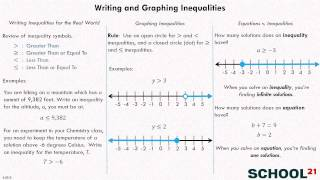By School 21# Graph systems of inequalities and check solutions

##By Khan Academy

Sal determines the solution set of the following system: yï¿½ï¿½ï¿½ï¿½ï¿½ï¿½ï¿½ï¿½ï¿½ï¿½ï¿½ï¿½ï¿½ï¿½ï¿½ï¿½ï¿½ï¿½ï¿½ï¿½ï¿½2x+1 and y<2x-5 and x>1. He does that by graphing the system and analyzing the graph.# Graph inequalities and check solutions

##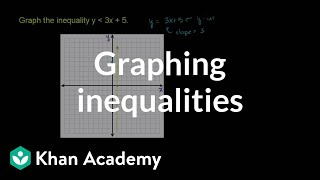By Khan Academy

Sal graphs the inequality y<3x+5.# Graph inequalities and check solutions

##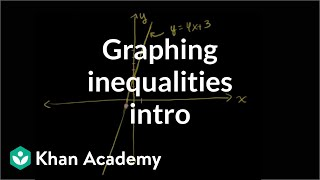By Khan Academy

Learn how to graph two-variable linear inequalities.# Graph systems of inequalities and check solutions

##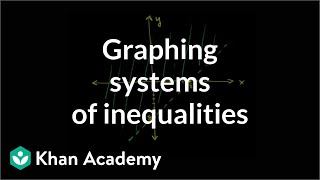By Khan Academy

Learn how to graph systems of two-variable linear inequalities.# Introduction to graphing inequalities | Two-variable linear inequalities | Algebra I | Khan Academy

##By Khan Academy

This video from Khan Academy demonstrates how to graph inequalities. Sal completes some example problems to show you how it's done.# Graphing Systems of Linear Inequalities - Example 2

##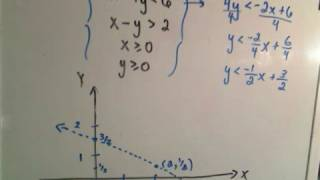By PatrickJMT

In this video, the instructor goes through the steps needed to graph a system of linear inequalities. He discusses slope, shows how to draw the line on the coordinate plane, and explains what section of the graph should be shaded. There is some shadow on the white board which makes it a little more difficult to see at times during the instruction.# Graphing Systems of Linear Inequalities - Example 2

##By PatrickJMT

In this video, the instructor goes through the steps needed to graph a system of linear inequalities. He discusses slope, shows how to draw the line on the coordinate plane, and explains what section of the graph should be shaded. There is some shadow on the white board which makes it a little more difficult to see at times during the instruction.# Graphing linear inequalities

##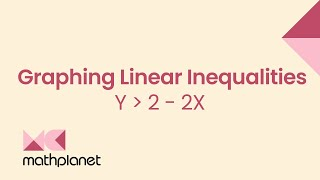By MathPlanetVideos

Graph the inequality y > 2 - 2x# Graphing inequalities 2 | Algebra Basics | Khan Academy

##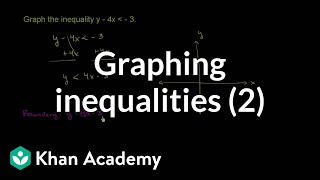By Khan Academy

Watch this example of graphing a strict linear inequality. Notice that the first step is converting the inequality into y < mx + b form.# One-step inequalities

##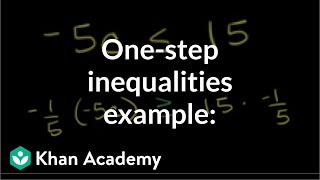By Khan Academy

In addition to solving the inequality, we'll graph the solution. Remember to swap if you mutiply both sides of the inequality by a negative number.# Graph inequalities

##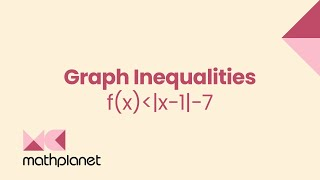By MathPlanetVideos

Graph f(x)# Check solutions of two-variable linear inequalities

##By Khan Academy

Sal determines whether the ordered pairs (3,5) and (1,-7) are solutions of the inequality 5x-3yï¿½ï¿½ï¿½ï¿½ï¿½ï¿½ï¿½ï¿½ï¿½ï¿½ï¿½ï¿½ï¿½ï¿½ï¿½ï¿½ï¿½ï¿½ï¿½ï¿½ï¿½25. He discussed the problem both algebraically and graphically.# Two-variable linear inequalities word problems

##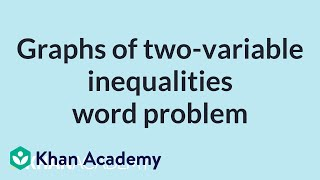By Khan Academy

Sal is given the graph of a two-variable linear inequality that models a context about dog biscuits! He analyzes it to learn about the context and to check a possible solution.# Absolute value inequalities | Linear equations | Algebra I | Khan Academy

##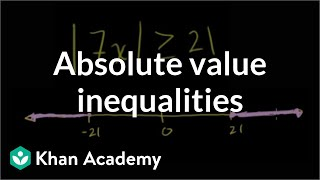By Khan Academy

This video lecture series on Worked Examples in Algebra from Khan Academy includes Solving Equations, Solving Word Problems, Solving for a variable, Absolute Value and Number Lines, Patterns in Sequences, Functional Relationships, Domain and Range, Rate Problems, Linear Functions, Slope of a Line, X and Y intercepts, Equation of a Line, Parallel Lines, Perpendicular Lines, Solving Inequalities and more...# Systems of linear inequalities word problems

##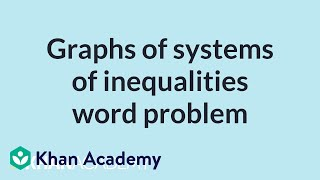By Khan Academy

Sal is given the graph of a two-variable linear inequality that models a context about chopping vegetables. He analyzes the solution set of the system in terms of the context.# Constraint solution sets of two-variable linear inequalities

##By Khan Academy

Sal determines which x-values make the ordered pair (x,-7) a solution of 2x-7y<25. He also solves a similar problem where the inequality is given as a graph.# Constraint solution sets of systems of linear inequalities

##By Khan Academy

Given the graph of a system of inequalities, Sal finds the x-values that make the ordered pair (x,-2) a solution of the system, which is the solution set constrained to y=-2. Then he solves a similar problem where x is constrained to 4.# Writing inequalities to describe real-world situations

##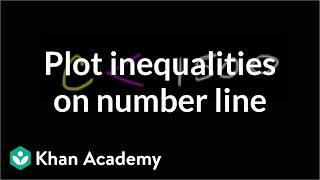By Khan Academy

Learn how to graph an inequality on a number line to indicate which values make it true.